# 07. LU Decomposition.pptx

May. 27, 2023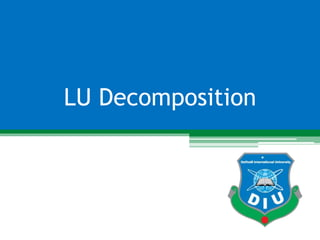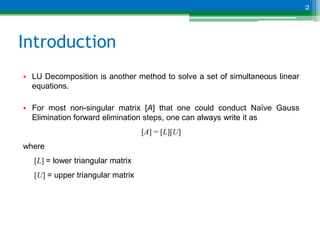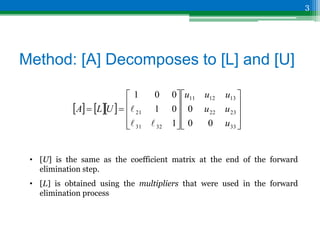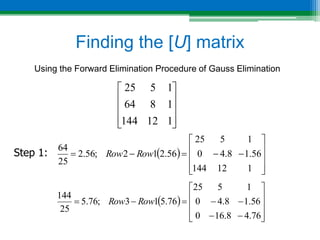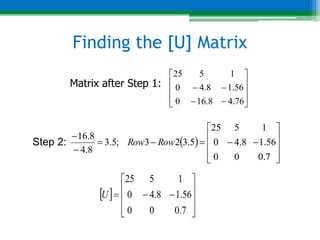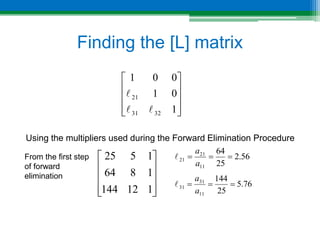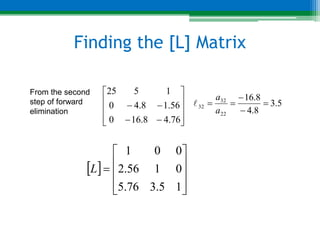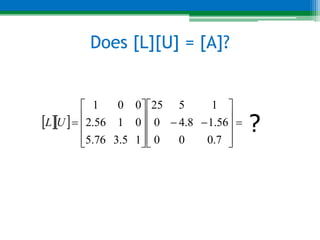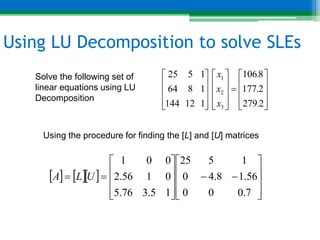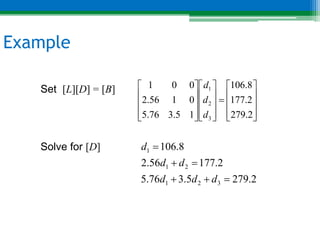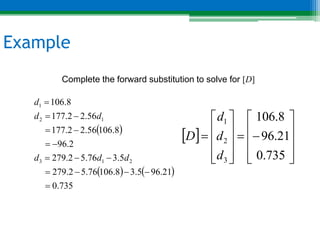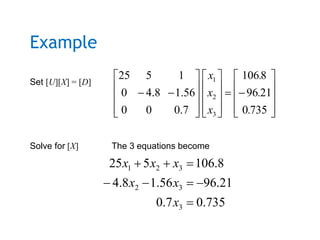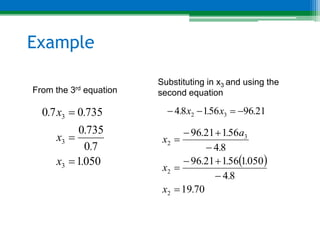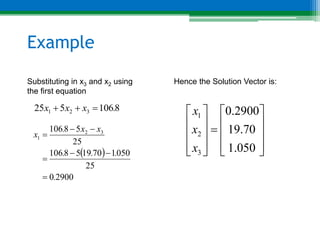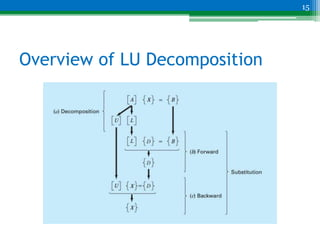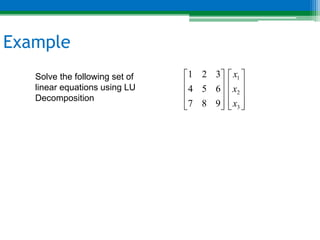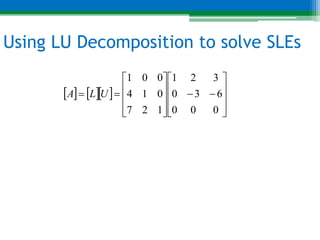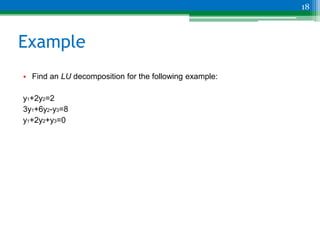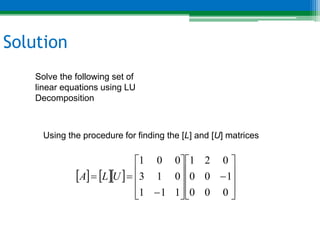1 of 20

### 07. LU Decomposition.pptx

• 1. LU Decomposition 1
• 2. Introduction • LU Decomposition is another method to solve a set of simultaneous linear equations. • For most non-singular matrix [A] that one could conduct Naïve Gauss Elimination forward elimination steps, one can always write it as [A] = [L][U] where [L] = lower triangular matrix [U] = upper triangular matrix 2
• 3. Method: [A] Decomposes to [L] and [U] 3                            33 23 22 13 12 11 32 31 21 0 0 0 1 0 1 0 0 1 u u u u u u U L A    • [U] is the same as the coefficient matrix at the end of the forward elimination step. • [L] is obtained using the multipliers that were used in the forward elimination process
• 4. Finding the [U] matrix Using the Forward Elimination Procedure of Gauss Elimination 1 12 144 1 8 64 1 5 25             1 12 144 56 . 1 8 . 4 0 1 5 25 56 . 2 1 2 ; 56 . 2 25 64                Row Row   76 . 4 8 . 16 0 56 . 1 8 . 4 0 1 5 25 76 . 5 1 3 ; 76 . 5 25 144                  Row Row Step 1:
• 5. Finding the [U] Matrix Step 2:               76 . 4 8 . 16 0 56 . 1 8 . 4 0 1 5 25   7 . 0 0 0 56 . 1 8 . 4 0 1 5 25 5 . 3 2 3 ; 5 . 3 8 . 4 8 . 16                  Row Row                7 . 0 0 0 56 . 1 8 . 4 0 1 5 25 U Matrix after Step 1:
• 6. Finding the [L] matrix Using the multipliers used during the Forward Elimination Procedure           1 0 1 0 0 1 32 31 21    56 . 2 25 64 11 21 21    a a  76 . 5 25 144 11 31 31    a a  From the first step of forward elimination 1 12 144 1 8 64 1 5 25          
• 7. Finding the [L] Matrix              1 5 . 3 76 . 5 0 1 56 . 2 0 0 1 L From the second step of forward elimination               76 . 4 8 . 16 0 56 . 1 8 . 4 0 1 5 25 5 . 3 8 . 4 8 . 16 22 32 32      a a 
• 8. Does [L][U] = [A]?                            7 . 0 0 0 56 . 1 8 . 4 0 1 5 25 1 5 . 3 76 . 5 0 1 56 . 2 0 0 1 U L ?
• 9. Using LU Decomposition to solve SLEs Solve the following set of linear equations using LU Decomposition                                2 279 2 177 8 106 1 12 144 1 8 64 1 5 25 3 2 1 . . . x x x Using the procedure for finding the [L] and [U] matrices                              7 . 0 0 0 56 . 1 8 . 4 0 1 5 25 1 5 . 3 76 . 5 0 1 56 . 2 0 0 1 U L A
• 10. Example Set [L][D] = [B] Solve for [D]                                2 . 279 2 . 177 8 . 106 1 5 . 3 76 . 5 0 1 56 . 2 0 0 1 3 2 1 d d d 2 . 279 5 . 3 76 . 5 2 . 177 56 . 2 8 . 106 3 2 1 2 1 1       d d d d d d
• 11. Example Complete the forward substitution to solve for [D]       735 . 0 21 . 96 5 . 3 8 . 106 76 . 5 2 . 279 5 . 3 76 . 5 2 . 279 2 . 96 8 . 106 56 . 2 2 . 177 56 . 2 2 . 177 8 . 106 2 1 3 1 2 1                d d d d d d                          735 . 0 21 . 96 8 . 106 3 2 1 d d d D
• 12. Example Set [U][X] = [D] Solve for [X] The 3 equations become                                   735 0 21 96 8 106 7 . 0 0 0 56 . 1 8 . 4 0 1 5 25 3 2 1 . . . x x x 735 . 0 7 . 0 21 . 96 56 . 1 8 . 4 8 . 106 5 25 3 3 2 3 2 1         x x x x x x
• 13. Example From the 3rd equation 050 1 7 0 735 0 735 0 7 0 3 3 3 . x . . x . x .    Substituting in x3 and using the second equation 21 96 56 1 8 4 3 2 . x . x .       70 19 8 4 050 1 56 1 21 96 8 4 56 1 21 96 2 2 3 2 . x . . . . x . a . . x         
• 14. Example Substituting in x3 and x2 using the first equation 8 106 5 25 3 2 1 . x x x    Hence the Solution Vector is:                      050 . 1 70 . 19 2900 . 0 3 2 1 x x x   2900 0 25 050 1 70 19 5 8 106 25 5 8 106 3 2 1 . . . . x x . x       
• 15. Overview of LU Decomposition 15
• 16. Example Solve the following set of linear equations using LU Decomposition                     3 2 1 9 8 7 6 5 4 3 2 1 x x x
• 17. Using LU Decomposition to solve SLEs                              0 0 0 6 3 0 3 2 1 1 2 7 0 1 4 0 0 1 U L A
• 18. Example • Find an LU decomposition for the following example: y1+2y2=2 3y1+6y2-y3=8 y1+2y2+y3=0 18
• 19. Solution Solve the following set of linear equations using LU Decomposition Using the procedure for finding the [L] and [U] matrices                              0 0 0 1 0 0 0 2 1 1 1 1 0 1 3 0 0 1 U L A
• 20. Thank You! 20